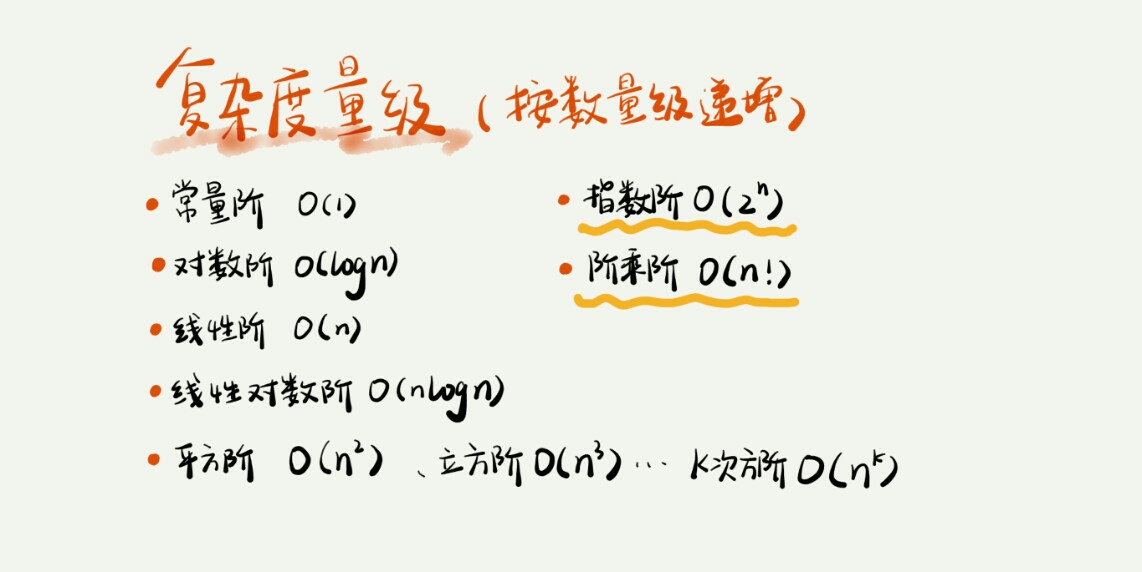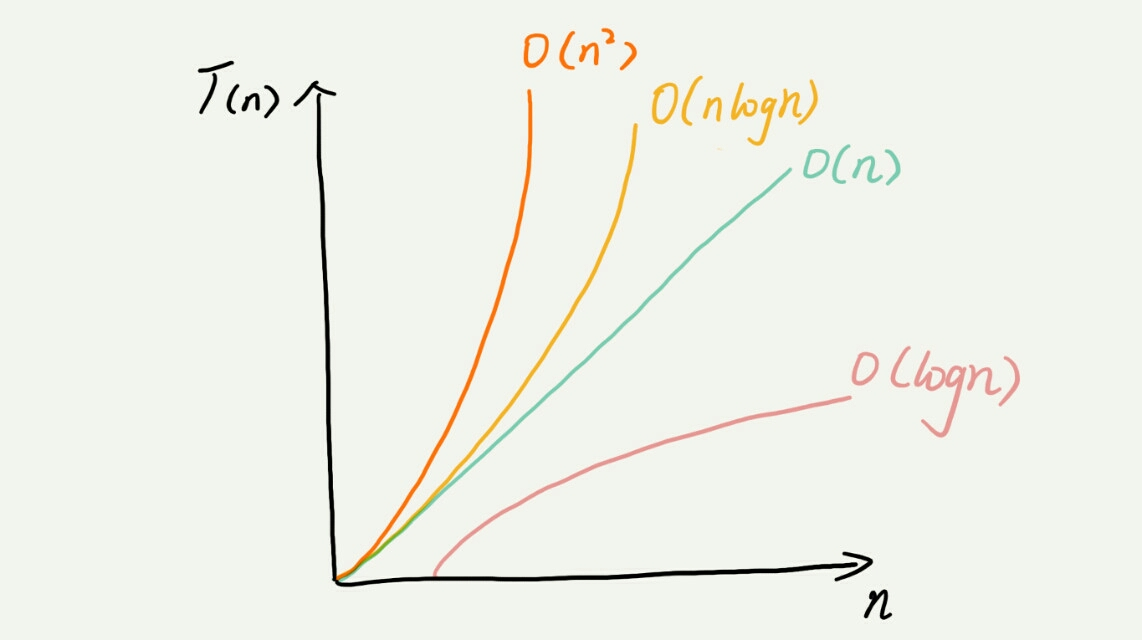# 复杂度分析(上)

@(数据结构与算法)

1. 数据结构与算法本质上是解决程序运行速度快存储空间省的问题，所以需要通过一个指标，即时间、空间复杂度来衡量这个问题
2. 为什么需要复杂度分析
• 程序测试运行结果会受到测试环境的硬件影响
• 测试结果受数据规模的影响很大
1. 假设每行代码的运行时间相同，则可得到所有代码的执行时间 T(n) 与每行代码的执行次数成正比。
2. 代码块：
1 int cal(int n){
2	int sum = 0;             	//执行 1 次
3	int i = 1:				 	//执行 1 次
4	int j = 1;				 	//执行 1 次
5	for(;i <= n;++i){		 	//执行 n 次
6		j = 1;				 	//执行 n 次
7		for(; j <= n; ++j){		//执行 n^2 次
8			sum = sum + i * j;	//执行 n^2 次
9		}
10	}
11 }


5. 大 $O$ 时间复杂度公式：
$$T(n) = O(f(n))$$

6. 分析代码时间复杂度的方法：

• 只关注循环执行次数最多的一段代码。
• 加法法则：总复杂度等于量级最大的那段代码的复杂度
• 乘法法则：嵌套代码的复杂度等于嵌套内外代码复杂度的乘积
1	int cal(int n){
2		int ret = 0;
3		int i = 1;
4		for (; i<n; ++i){
5		ret = ret + f(i)
6		}
7	}
8
9	int f(int n){
10		int sum = 0;
11		int i = 1;
12		for(; i < n; ++i){
13			sum = sum + i;
14		}
15		return sum;
16	}


7. 常见的时间复杂度量级：8. 空间复杂度：类比一下时间复杂度，表示的算法的空间与数据规模之间的增长关系。

1 void print(int n) {
2  int i = 0;
3  int[] a = new int[n];
4  for (i; i <n; ++i) {
5    a[i] = i * i;
6  }

7  for (i = n-1; i >= 0; --i) {
8    print out a[i]
9  }
10}posted @ 2018-09-26 17:55  我不  阅读(910)  评论(0编辑  收藏